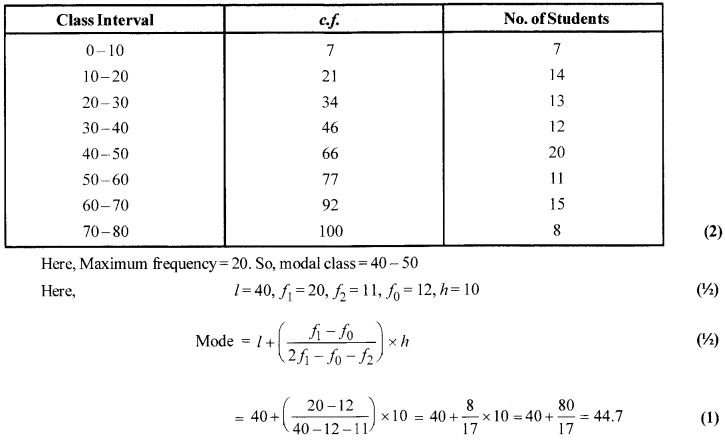# CBSE Sample Paper for Class 10th Mathematics Paper 08 | CBSE Exam | CBSE Notes

Here we are giving sample papers to all of you CBSE board students, through which all of you can score very well in your exams. In this post, we are giving you a sample paper of class 10 maths. hope you benefit from it. You can download the pdf file of this sample paper. CBSE sample paper 2021 class 10 and cbse sample paper 2021 class 12

## CBSE Sample Paper for Class 10th Mathematics Paper 08 | CBSE Exam | CBSE Notes

 Board CBSE Class X ( 10th ) Subject Mathematics Sample Paper No. Paper 08 Category CBSE Sample Paper

Students who are going to appear for CBSE Class 10 Examinations are advised to practice the CBSE sample papers given here which is designed as per the latest Syllabus and marking scheme, as prescribed by the CBSE, is given here. Paper 1 of Solved CBSE Sample Paper for Class 10 Maths is given below with free pdf download solutions.Time allowed: 3 Hours
Maximum Marks: 80

General Instructions

• All questions are compulsory.
• The question paper consists of 30 questions divided into four sections A, B, C and D.
• Section A contains 6 questions of 1 mark each. Section B contains 6 questions of 2 marks each. Section C contains 10 questions of 3 marks each. Section D contains 8 questions of 4 marks each,
• There is no overall choice. However, an internal choice has been provided in four questions of 3 marks each and three questions of 4 marks each. You have to attempt only one of the alternatives in all such questions.
• Use of calculators is not permitted.

(Section-A)

Question 1.
Find the smallest positive rational number by which [latex s=2]\frac { 1 }{ 7 } [/latex] should be multiplied so that its decimal expansion terminates after 2 places of decimal.

Question 2.
If the vertices of a triangle are (1,2), (4, -6) and(3, 5) then, find the area of the triangle (NTSE2014,2015)

Question 3.
If [latex s=2]\left( \tan { \theta } +\frac { 1 }{ \tan { \theta } } \right) [/latex] = 2, then find the value of [latex s=2]\tan ^{ 2 }{ \theta } +\frac { 1 }{ \tan ^{ 2 }{ \theta } } [/latex](NTSE 2015)

Question 4.
Evaluate: $\sqrt { 6+\sqrt { 6 } +\sqrt { 6+ } }$…..

Question 5.
What is the common difference of an A.P. in which a21 -a7 = 84?

Question 6.
The areas of two similar triangles are 121 cm2 and 64 cm2 respectively. If the median of the first triangle is
12.1 cm, find the corresponding median of the other.

(Section-B)

Question 7.
Two bills of ₹ 6075 and ₹ 8505 respectively are to be paid separately by cheques of same amount. Find
the largest possible amount of each cheque.

Question 8.
Find the value of70 + 68 + 66 +……..+ 40.

Question 9.
Find the chance that a non-leap year contains 53 Saturdays.

Question 10.
Ajar contains 54 marbles each of which is blue, green or white. The probability of selecting a blue
marble and a green marble at random from the jar is [latex s=2]\frac { 1 }{ 3 } [/latex] and [latex s=2]\frac { 4 }{ 9 } [/latex] respectively. How many white marbles does the jar contain?

Question 11.
If the lines given by 3x + 2ky = 2 and 2x+5y= 1 are parallel, then find the value of k

Question 12.
Find ‘k’ so that the points (7, -2), (5,1) and (3, k) are collinear.

(Section-C)

Question 13.
If a2 – b2 is a prime number, show that a2 – b2 = a + b, where a, b are natural numbers.

Question 14.
If the polynomial 6x4 + 8x3 + 17x2 + 21x + 7 is divided by another polynomial 3x2 + 4x+ 1, the remainder comes out to be (ax + b ), find the values of a and b.

Question 15.
If tan θ + sin θ = m and tan θ – sin θ = n, then prove that m2 – n2 = 4$\sqrt { mn }$
OR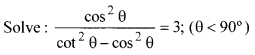Question 16.
The following table gives the number of pages written by Sarika for completing her book for 30 days: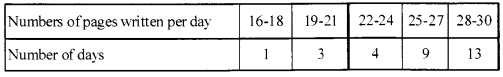Find the mean number of pages written per day.

Question 17.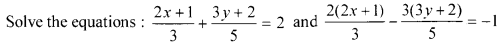Question 18.
A circle touches all four sides of a quadrilateral ABCD. Prove that AB + CD = BC + DA.

Question 19.
In figure, two concentric circles with centre O, have radii 21 cm and 42 cm. If ZAOB = 60°, find the area of the shaded region [ Use π = [latex s=2]\frac { 22 }{ 7 } [/latex] ].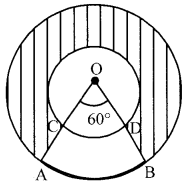Question 20.
If volumes of two spheres are in the ratio of 64 : 27, then what is the ratio of their surface areas?
OR
A medicine capsule is in the shape of a cylinder of diameter 0.5 cm with two hemispheres stuck to each of its ends. The length of the entire capsule is 2 cm. What is the capacity of the capsule?

Question 21.
If A is the area of a right angled triangle and ‘b’ is one of the sides containing the right angle, prove that
the length of the altitude on the hypotenuse is [latex s=2]\frac { 2Ab }{ \sqrt { { b }^{ 4 }+4{ A }^{ 2 } } } [/latex]
OR
ABC is a right. A right-angled at B. AD and CE are the two medians drawn from A and C respectively. If
AC = 5 cm and AD = [latex s=2]\frac { 3\sqrt { 5 } }{ 2 } [/latex] cm, find CE

Question 22.
If the points A (k + 1,2k), B (3k, 2k+ 3) and C (5k- 1,5k) are collinear, then find the value of k.
OR
Show that the points A (1, 0), B (5,3), C (2,7) and D (-2,4) are the vertices of a parallelogram.

(Section-D)

Question 23.
An aeroplane left 30 minutes later than its scheduled time. The pilot decided to increase its speed. To reach its destination 1500 km away in time, the pilot has to increase its speed by 250 km/hr from its usual speed. Determine the usual speed.

Question 24.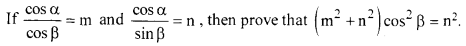Question 25.
Draw a line segment AB of length 8 cm. Taking A as the centre, draw a circle of radius 4 cm and taking B as centre, draw another circle of radius 3 cm. Construct tangents to each circle from the centre of the other circle.

Question 26.
If h, c, v are respectively the height, the curved surface area and the volume of a cone, prove that 3πvh3– c2h2+ 9v2=0

Question 27.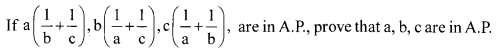Question 28.
If a line is drawn parallel to one side of a triangle to intersect the other two sides in distinct points, then prove that the other two sides are divided in the same ratio.
OR
If a line divides any two sides of a triangle in the same ratio, then prove that the line is parallel to the third side.

Question 29.
From the top of a tower, the angles of depression of two objects on the same side of the tower are found to be α and β ( α > β). If the distance between the objects is P; show that the height ‘h’ of the tower is given by h = [latex s=2]\frac { P\tan { \alpha } \tan { \beta } }{ \tan { \alpha } -\tan { \beta } } [/latex] .
OR
A vertical tower stands on a horizontal plane and is surmounted by vertical flag staff of height ‘h’ At a point on the plane, the angles of elevation of the bottom and the top of the flag- staff are α and β respectively. Prove that the height of the tower is [latex s=2]\frac { h\tan { \alpha } }{ \tan { \beta -\tan { \alpha } } } [/latex]

Question 30.
The weekly expenditure of5000 families are tabulated below :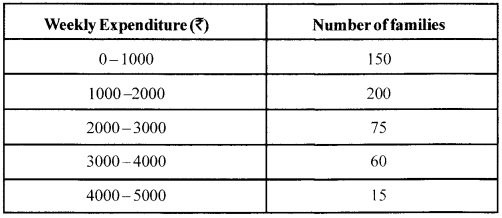Find the median expenditure.
OR
The following table shows the marks obtained by 100 students of class X in a school during a particular academic session. Find the mode of this distribution.Solutions
(Section-A)

Solution 1.
Since: [latex s=2]\frac { 1 }{ 7 } [/latex] × [latex s=2]\frac { 7 }{ 100 } [/latex] = [latex s=2]\frac { 1 }{ 100 } [/latex] = 0.01
The smallest rational number is [latex s=2]\frac { 7 }{ 100 } [/latex] (1)

Solution 2.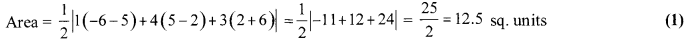Solution 3.Solution 4.
Let x = $\sqrt { 6+\sqrt { 6 } +\sqrt { 6+ } }$ ….Then, x = $\sqrt { 6+x }$
On squaring both the sides, we get
x2 = 6+ x ⇒x2-x-6 = 0
⇒ (x-3)(x + 2) = 0 ⇒ x = 3 or x = -2.
∵ x > 0
So, x = 3 (1)

Solution 5.
Since, a21 – a7 = 84 …(i)
Let the first term and common difference of
given A.P. be a and d respectively.
So, a21 = a+ (21 – 1)d = a + 20d
Now, a7= a + (7 – 1)d = a + 6d
From equation (i),
(a + 20d) – (a + 6d) = 84
⇒ (20-6)d= 84 ⇒ 14d= 84 ⇒d = 6
Hence, the common difference of given A.P. is 6. (1)

Solution 6.
Let ABC and DEFbe two triangles such that ∆ABC ~ ∆DEF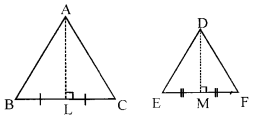Let AL, DM be their medians respectively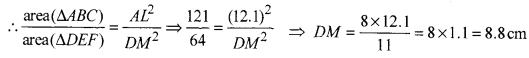Hence required corresponding median = 8.8 cm.

(Section-B)

Solution 7.
The largest possible amount of cheque will betheHCF (6075,8505).
Applying Euclid’s division lemma to 8505 and 6075, we have,
8505 = 6075 × 1 +2430 (1/2)
Since remainder 2430 ≠ 0 again applying division lemma to 6075 and 2430
6075 = 2430 × 2+1215 (1/2)
Again remainder 1215 ≠ 0
So, again applying the division lemma to 2430 and 1215
2430=1215 × 2 +0 (1/2)
Here the remainder is zero
So,H.C.F= 1215 (1/2)
Therefore, the largest possible amount of each cheque will be 1215.

Solution 8.
Series: 70 + 68 + 66 + +40
Here a = 70, d = 68 – 70 = -2, tn = 1 = 40.
Now, tn = a + (n-1)d =70 + (n-1)(-2) ⇒ 40 = 70 – 2n + 2 ⇒n= 16 (1)
∴ Sn =[latex s=2]\frac { n }{ 2 } [/latex][a + l] ⇒Sn = [latex s=2]\frac { 16 }{ 2 } [/latex][70 + 40] = 880 (1)

Solution 9.
S = {S, M, T, W, Th, F, Sa} (1/2)
n(S)=7
A non-leap year contains 365 days,
i.e., 52 weeks + 1 day.
E={Sa}
n(E)=1 (1/2)
∴ P(E) = [latex s=2]\frac { n(E) }{ n(S) } [/latex] = [latex s=2]\frac { 1 }{ 7 } [/latex] (1)

Solution 10.
Let there be x blue, y green and z white marbles in the jar.
Then, x+y+ z=54 …(i) (1/2)
∴ P(selecting a blue marble) = [latex s=2]\frac { x }{ 54 } [/latex] ⇒[latex s=2]\frac { x }{ 54 } [/latex] = [latex s=2]\frac { 1 }{ 3 } [/latex] ⇒ x = 18 (1/2)
Similarly, /’(selecting a green marble) = [latex s=2]\frac { y }{ 54 } [/latex] ⇒ [latex s=2]\frac { y }{ 54 } [/latex]= [latex s=2]\frac { 4 }{ 9 } [/latex] ⇒y = 24 (1/2)
Substituting thevaluesofxandyin(i),z= 12
Hence, the jar contains 12 white marbles. (1/2)

Solution 11.
Given lines,
3x + 2ky- 2 = 0
and 2x+ 5y-1 =0
Here, a1 =3,b1 =2k, c1 =-2
a2 = 2, b2 = 5, c2 =-1 (1)
Condition for parallel lines is [latex s=2]\frac { 3 }{ 2 } [/latex] = [latex s=2]\frac { 2k }{ 5 } [/latex] ⇒k = [latex s=2]\frac { 15 }{ 4 } [/latex] (1)

Solution 12.
Since points are collinear,
so, x1(y2 – y3) + x2(y3 – y1) + x3(y1 – y2) = 0 (1/2)
Taking (7, -2) as (x1, y1), (5,1) as (x2, y2) and (3, k) as (x3, y3), we have
7(1 – k) + 5(k + 2) + 3 (-2 -1) = 0 (1/2)
or, 7 – 7k + 5k + 10 – 9 = 0 ⇒ 2k = 8 ⇒k = 4. (1)

(Section-C)

Solution 13.
a2 – b2 = (a-b)(a +b) ….(1)
∵ a2 -b2 is a prime number (1)
∴One of the two factors = 1
So, a — b= 1 [∵a – b< a+b]
∵ The only divisors of a prime number are 1 and it self. (1)
(1) become a2 -b2= 1 (a + b)
or a2 – b2 = a + b
e.g., 32 – 22 = 5 (which is prime)
⇒ 32 – 22 = 3 +2 (1)

Solution 14.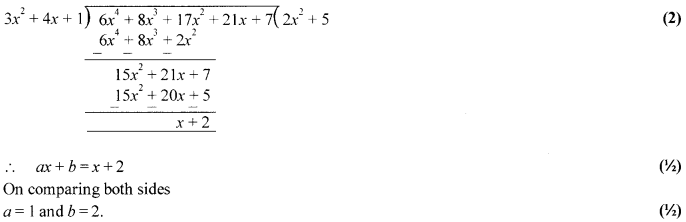Solution 15.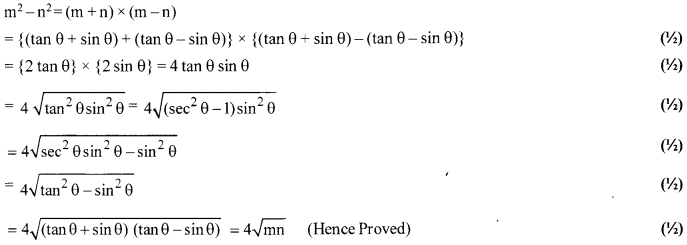OR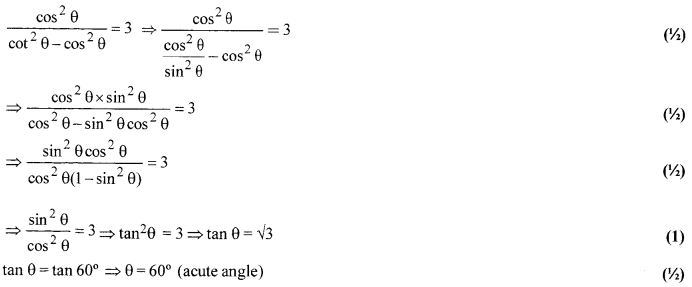Solution 16.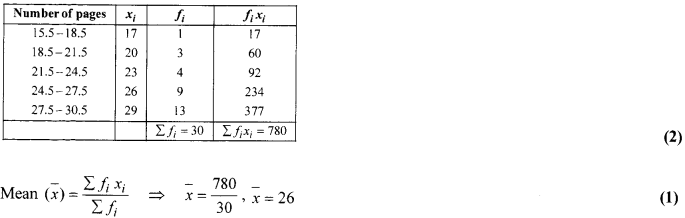Solution 17.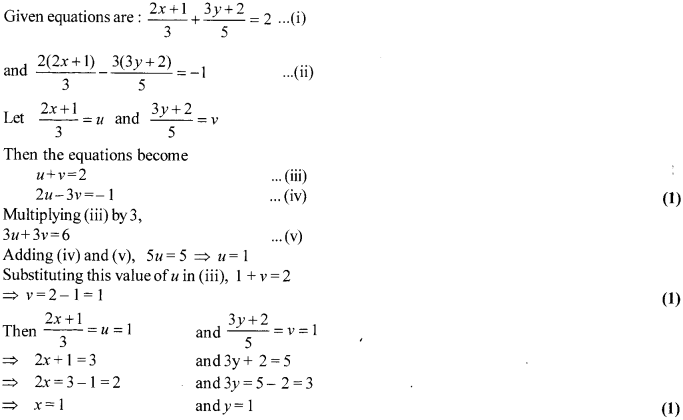Solution 18.
Let there be a circle with centre O whereas AB, BC, CD and DA are tangents at P, Q, R and S respectively.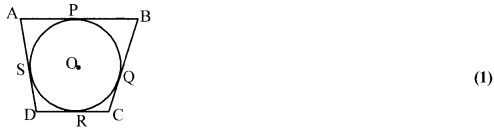Here,
AP = AS …(i)
BP = BQ …(ii)
CR=CQ …(iii)
DR=DS …(iv)
[Tangents drawn from a point (outside the circle) on a given circle are equal in lengths]
From equations (i), (ii), (iii) and (iv), (1)
(AP +BP) + (CR + DR) = (BQ + CQ) + (DS + AS)
AB + CD = BC + DA (Hence proved.) (1)

Solution 19.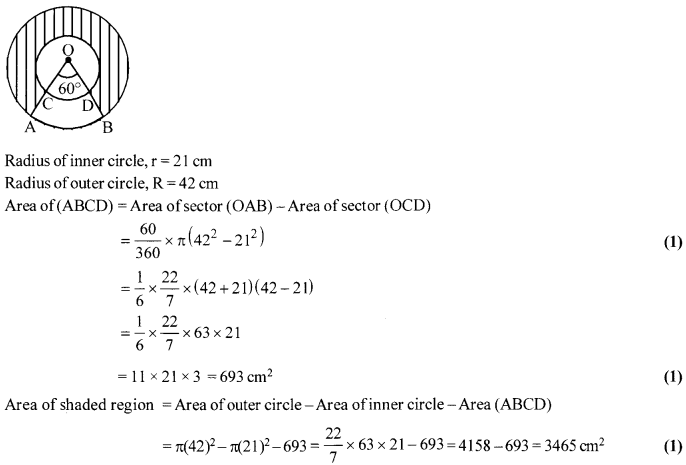Solution 20.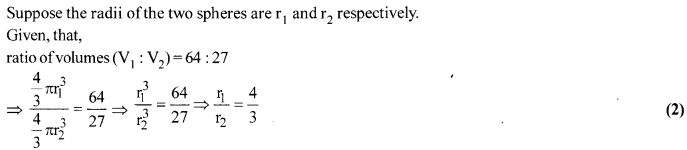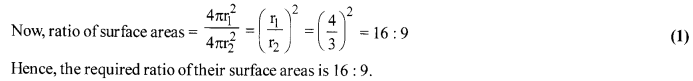OR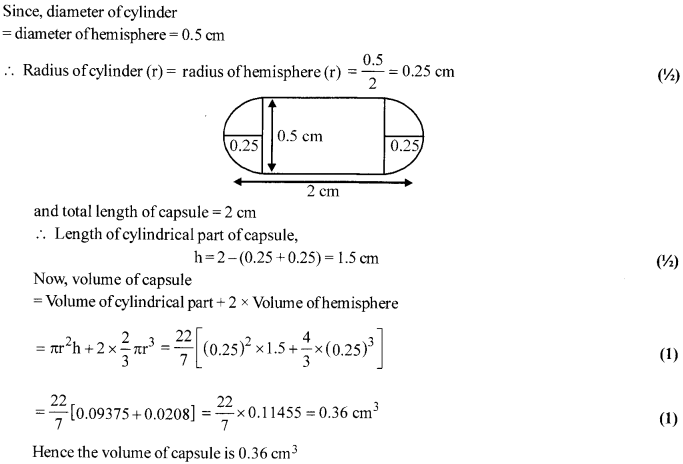Solution 21.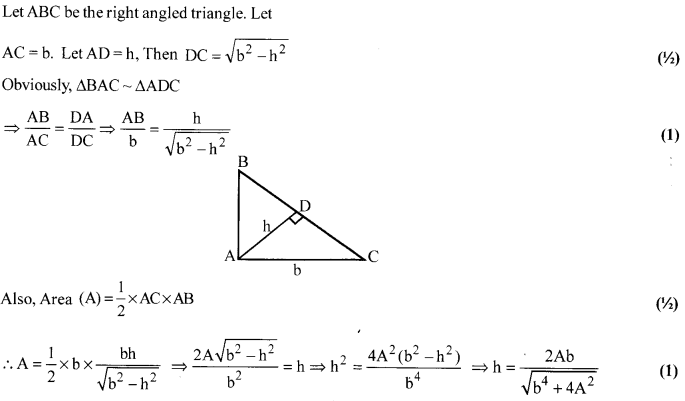OR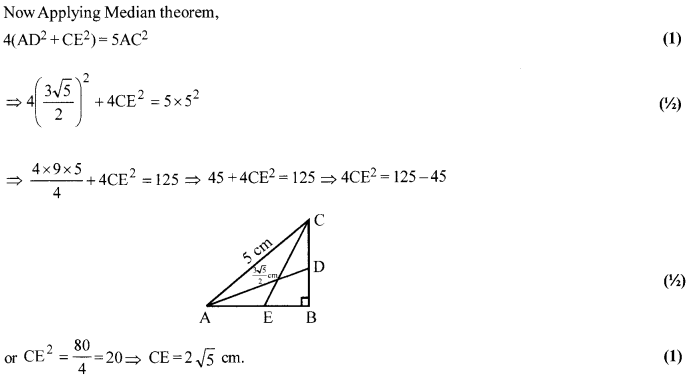Solution 22.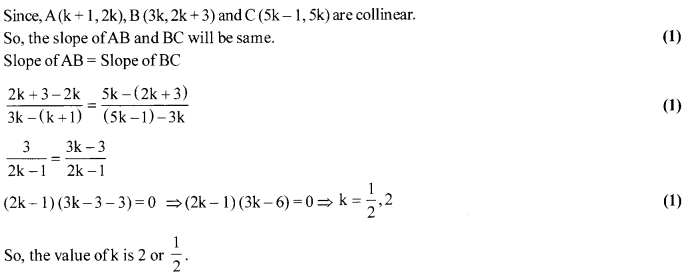OR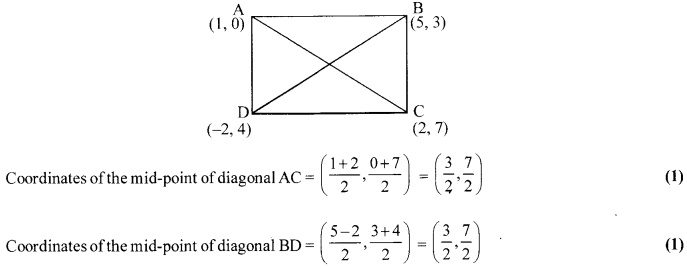Since the coordinates of the mid-points of diagonals AC and BD are same.
∴ They bisect each other.
Hence, ABCD is a parallelogram. (1)

(Section-D)

Solution 23.Solution 24.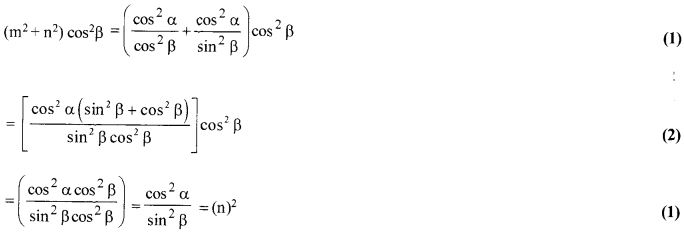Solution 25.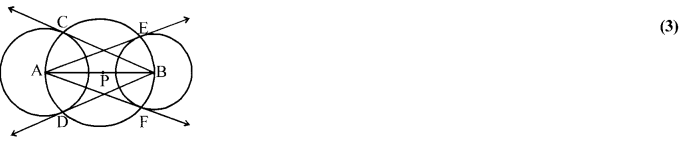Steps of construction:
(i) Draw a line AB of length 8 cm.
(ii) With centre A and radius 4 cm, draw a circle, which intersect AB at point P.
(iii) With centre B and radius 3 cm, draw the second circle.
(iv) With centre P and radius PA or PB draw the third circle, which intersects the circle drawn in step (ii) at C & D and the circle drawn in step (iii) at E & F.
(v) Draw rays AE,AF,BC and BD.
(vi) Thus AE, AF, BC and BD are required tangents. (1)

Solution 26.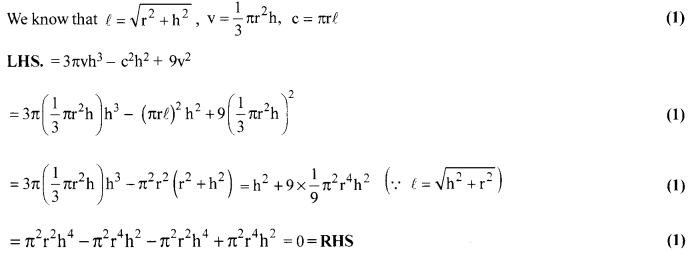Solution 27.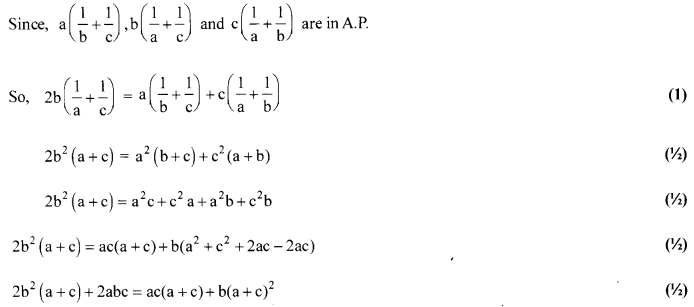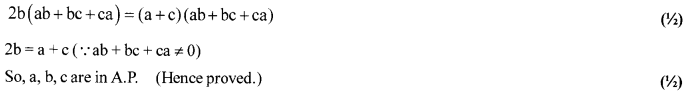Solution 28.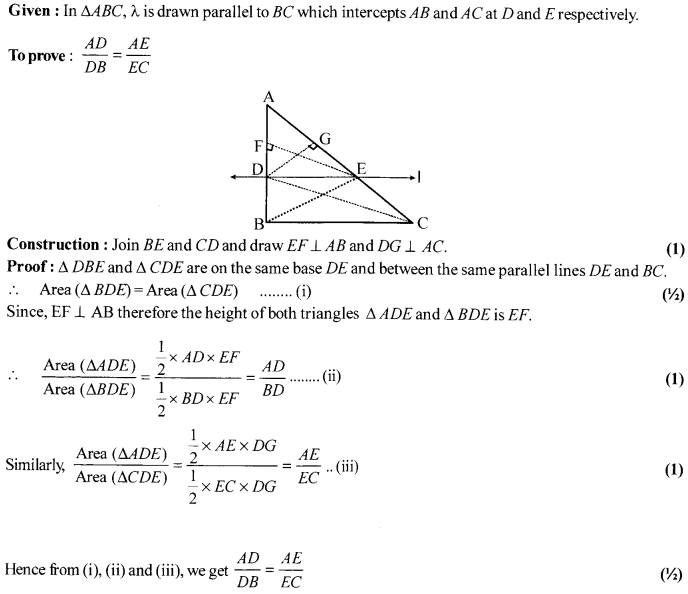OR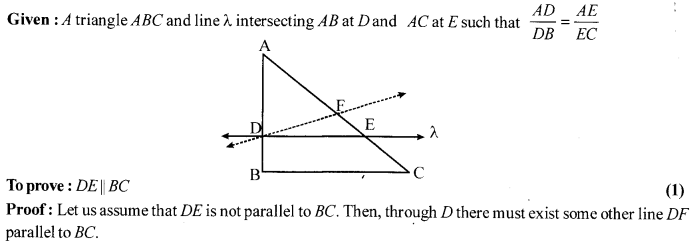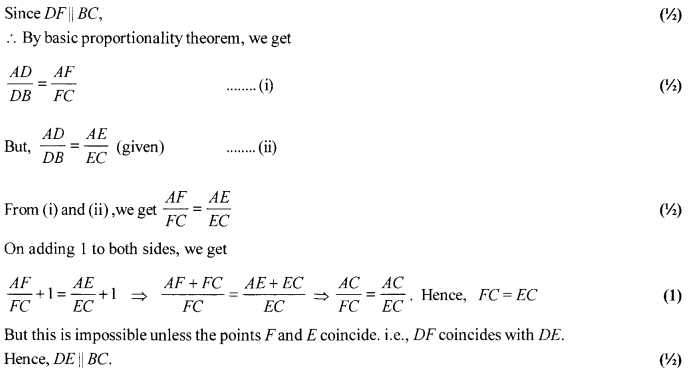Solution 29.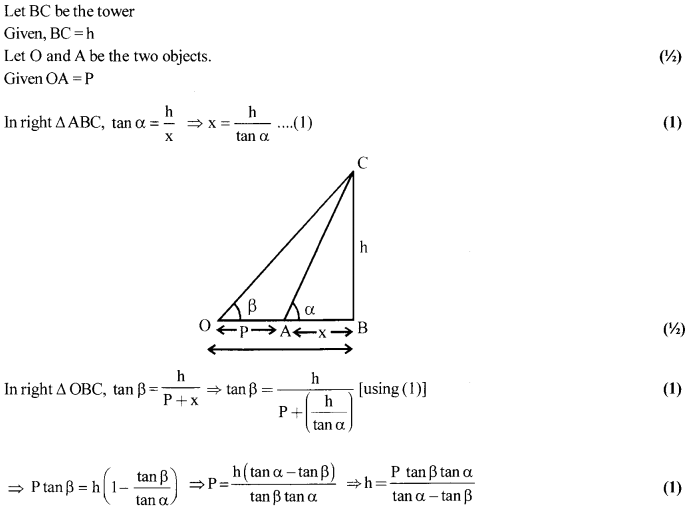OR
Let AB be the tower and BC be the flag.
LetAB = H
BC = h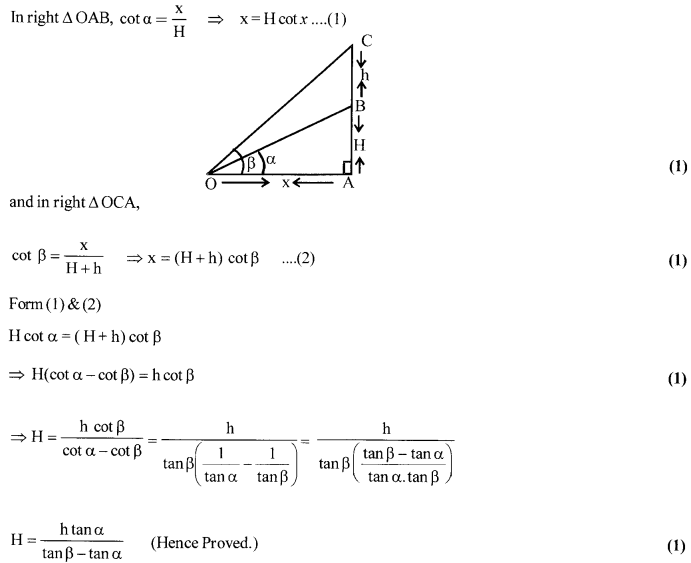Solution 30.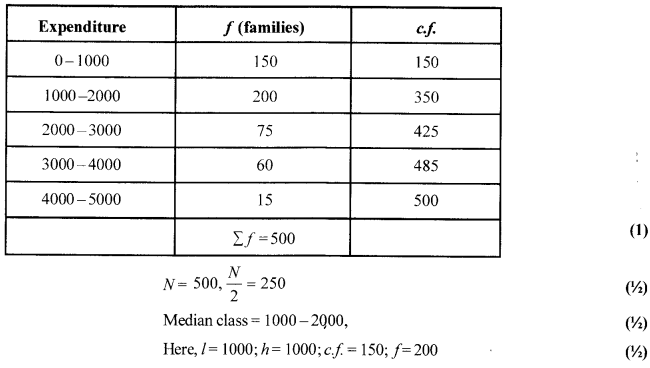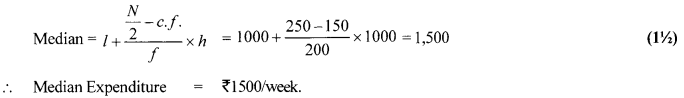OR